# Physical Approach for Sand Flux Quantification and Flow Dynamic Properties Investigation for Fine Sand Grains Transport

Physical Approach for Sand Flux Quantification and Flow Dynamic Properties Investigation for Fine Sand Grains Transport

F-Zahra BenarabAhmed Medjelled Toufik Benchatti

Laboratory of mechanics, University Amar Telidji, Laghouat 03000, Algeria

Corresponding Author Email:
f.benarab@lagh-univ.dz
Page:
671-676
|
DOI:
https://doi.org/10.18280/ijht.340417
|
Accepted:
|
Published:
31 December 2016
| Citation

OPEN ACCESS

Abstract:

The purpose of this work is to study sand grains transport for the saltation mode. A numerical simulation approach is adopted with the introduction of a density distribution function. That aims to quantify the transported sand grains, according to the continuum theory. Making a prediction model for transported quantities. In this study, a fine sand grain size is taken into consideration as d=0. 295mm. The inlet wind velocities vary between 8 &18 m/s. The simulation results of sand flux are validated using previous experimental results. The mean horizontal velocity profiles are shown and discussed. It is noted that the sand flux can be described as a Gaussian function which is in good agreement with the experimental results in tunnels for the fine sand groups. This results lead to deduce the lift-off velocity and incident angles profiles distribution. From the sand-coupled wind velocity profile; it can be found that the wind velocity profile near to the surface is modified from logarithmic to a linear function. The variation of the turbulent kinetic energy with increasing wind velocity is further discussed. Finally the energy dissipation is shown. This model will find application in studies of saltation mode on both Earth and Mars.

Keywords:

Aeolian transport, Saltation, Transport layer, Sand flux, Turbulence kinetic energy.

1. Introduction

Aeolian sand transport is an essential process in natural environments such as desert areas, sandy beaches and other planets . It causes wind erosion and desertification in arid and semi-arid regions . Changes in earth surface topography through the formation of sand dune and ripples  At a large scales modifying radioactive transfers in the atmosphere, impacting cloud and precipitation formation. Impact in weather changes have been observed in the second half of 20th century. The lack of rain, wind erosion and displacement of soil led to: degradation in agricultural land , cultivated organic soil, sandy coastal areas, alluvial soil along rivers and other areas . Aeolian sand transport has a negative effect on air quality by reducing atmospheric visibility; impede traffic, damaging crops and electric lines and recently the degradation of solar panels . According to , saltation is the dominant mode of blown sand movement, accounting for about 75% of the total sand flux. In saltation sand grains are dislodged from the ground by turbulence and driven by wind. Wind accelerates sand grains that hop along the surface.  As its mass is big enough, the grain ends up falling with great kinetic energy and that will help in moving other grains. A sand cloud appears, kind of mixture between air and the flying grains with varying density, so the transported sand is more prominent near to the bed and it decreases with height.  Recently, the erodible grains can be separated on two groups: fine sand groups (0.063–0.8 mm) and coarse sand groups (0.8–2.0mm) according to .

In order to explore the fundamental internal mechanism in aeolian sand transport, numerical and analytical studies have been carried out generally subdivided into several different sub-processes –. They attempt to understand and quantifying the horizontal mass flux of saltating particles following wind intensity, with soil particles size and ground characteristics . Eulerian approaches have been developed as alternative and complementary to the Lagrangian approach in which the features of the particle and fluid phases are coupled and the resolution of the problem is not straightforward . Eulerian approaches are basically interested on the description of particle phase as a continuum characterized by a saturated density and an averaged velocity of the saltation . They do not require on explicit calculation of the particles trajectories. The recent continuum approaches developed by – parameterize the large distribution of particles velocities. Further approaches for better parameterizing the particle velocity distribution is to consider two effective species of reptating and saltating grains.

A proposal of the development of an effective continuum model is presented in this paper. A numerical simulation is worked out with the introduction of a density distribution function. That aims the quantification of transported sand grains. The wind velocity profile is calculated; it’s found that it follow a linear law in the Transport Layer. The turbulent kinetic energy variation during the transport with the increasing height is calculated too for growing wind velocities. A direct comparison between the sand flux and the turbulent kinetic energy is represented to trace the behavior of and clarify the relationship between the two curves. Finally, to explain that more, the energy dissipation is thoroughly discussed.

2. Mathematical Model

The mathematical model that governs such turbulent and incompressible flows consists of the continuity equation Eq(1), the Reynolds Averaged Navier-Stokes equations Eq(2), and the equations of turbulence model used (The standard κ-ε model), Eqs(3-4) .

$\frac{\delta \rho U_{i}}{\delta t}+\frac{\delta \rho U_{i}}{\delta x_{i}}=0$(1)

$\frac{\delta \rho U_{i}}{\delta t}+\frac{\delta \rho U_{i} U_{j}}{\delta x j}=\frac{-\delta P}{\delta x_{j}}+\frac{\delta}{\delta x_{j}}\left(\mu S_{i j}-\overline{u_{i}^{\prime} u_{j}^{\prime}}\right)$(2)

$\frac{d k}{d t}=\frac{\delta}{\delta x_{j}}\left(\frac{\mu_{t}}{\sigma_{k}} \frac{\delta k}{\delta x_{j}}\right)+P_{k}-\rho \varepsilon$(3)

$\frac{d k}{d t}=\frac{\delta}{\delta x_{i}}\left(\frac{\mu_{t}}{\sigma_{\varepsilon}} \frac{\delta \varepsilon}{\delta x_{i}}\right)+c_{\varepsilon 1} \frac{\varepsilon}{\kappa} P_{k}-\rho c_{\varepsilon 2} \frac{\varepsilon^{2}}{\kappa}$(4)

where, $S_{i j}$ is the mean strain-rate tensor by $S_{i j}=\frac{1}{2}\left(\frac{\delta U_{i}}{\delta x_{j}}+\frac{\delta U_{j}}{\delta x_{i}}\right)$ and $\overline{u_{i}^{\prime} u_{j}^{\prime}}=\mu_{t} S_{i j}-\frac{2}{3} k \delta_{i j}$

where, $k=\frac{1}{2} \overline{u_{i}^{\prime} u_{j}^{\prime}}$ is the turbulent kinetic energy  Is the Kronecker delta, ε is the dissipation of kinetic turbulent energy, μ is the kinetic viscosity, μt  denotes the turbulent kinematic viscosity and Pκ is the net production per unit dissipation of the turbulent kinematic energy; However:

$\mu_{t}=\rho c_{\mu} \frac{k^{2}}{\varepsilon}, P_{k}=\mu_{t} \frac{\delta U_{i}}{\delta x_{j}} S_{i j}$

According to the literature the empirical constraints of the turbulence model (standard  κ-ε) are given hereafter :

$c_{\mu}=0.09, c_{s 1}=1.44, c_{s 2}=1.92, \sigma_{\varepsilon}=1.3, \sigma_{k}=1$

3. Simulation Model and Boundary Conditions

In order to validate the results with those of , some conditions and hypothesis were taken:

• The computational domain of the flow field is about 12 m long, 1.3m wide and 1.45 m high.
• The grains diameter taken is 0.295mm.
•  The wind velocities vary between 8 and 18 m/s.
• Air is treated as incompressible since Mach number is less than 0.05.

Accordingly, the boundary conditions can be written as follows:

Bottom boundaries:

$u=u_{*}, v=0, w=0$

Top boundaries:

$\frac{\delta u}{\delta x}=0, v=0, w=0$

Outlet:

$\frac{\delta u}{\delta x}=0, \frac{\delta v}{\delta y}=0, \frac{\delta w}{\delta z}=0$

At the inlet, a logarithmic function is taken for the wind velocity profile :

$U(z)=\frac{u_{*}}{K} \log \frac{z}{z_{0}}$        $z \geq z_{0}$        (5)

where U (z) represents the velocity at height z,  $u_{*}$ is the friction velocity, k is the constant of Karman (0.41) and z0 is the aerodynamic roughness height of the surface .

According to the experimental studies, the aerodynamic roughness length can be calculated from the following equation, where d is the grain diameter:

$z_{0}=\frac{d}{30}$

Using the results of experimental works –; and starting from the relation between flux and density given by :

$q=\rho_{s} U(z)$(6)

It’s noticed that the concentration of sand in a sand-air mixture can be described by an exponential function.

$C=a e^{-z b}$(7)

where a and b are respectively the stream-wise mass dimensionless parameter of the density and the length scale parameter. Both a and b change regularly with the velocity, grain diameter and with height. We note that the parameter b is also a function of the horizontal length covered:

$a\left(z, d, u_{*}, d\right)=\frac{z_{0 s} \sqrt{g d}}{\beta U(z)}$

$b\left(x, z, d, u_{*}\right)=\left(\frac{U(z)}{x \sqrt{g d}}\right)$

Hence the quantity of sand transported can be expressed by:

$\rho_{s}=\rho_{s o u d} C$(8)

The density of the mixture will take the following form:

$\rho=\rho_{s a n d} C+\rho_{a i r}$(9)

With $Z_{0 s}$ is the roughness length saltation: $z_{0 s}=\alpha \frac{u_{*}^{2}}{g}$

With the coefficient α found by , α=0.02 $\rho_{\mathrm{air}}=1.25 \mathrm{kg} / \mathrm{m}^{3}, \rho_{\mathrm{sand}}=2650 \mathrm{kg} / \mathrm{m}^{3}$ and $\beta=0.6 \mathrm{m}$, which corresponds to the drag length establish by .

The governing equations (1-4) are solved using the finite-volume method with the centered discretization schemes at the cells boundary approximation. The 2nd order algorithm was used for pressure-velocity coupling; a transient implicit scheme is solved with the 2nd order Backward Euler.

4. Rusults and Discussion

The simulation calculation results are compared with the experimental results of  for the validation of the presented model. For this purpose, the calculated values of velocities and sand fluxes are taken at 8.5m from the test section entrance. Figure 1 shows the wind velocity profiles at the test section entrance calculated by Eq(5) compared to the velocity measured by .

The friction velocities corresponding to the four velocities (8, 10, 12 and 14 m/s) are respectively: 0.34, 0.415, 0.488 and 0.553 m / s.

## 1.png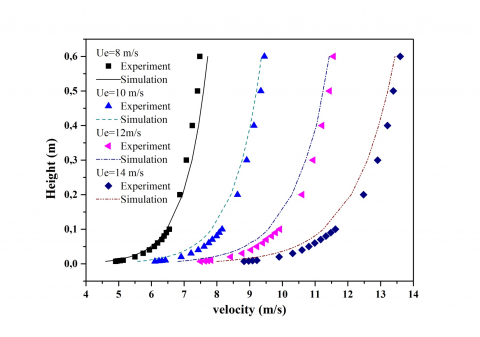Figure 1. The wind velocity profiles at the tunnel entrance

## 2.png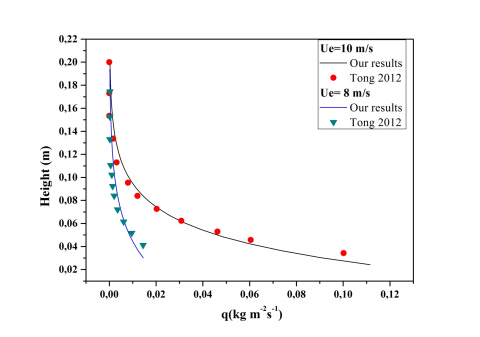Figure 2. Variation of sand flux with increasing height

Figure 2 and Figure 3 respectively represent the sand flux at various heights and mass flux for the deferent friction velocities. The comparison with the results of and  shows that the results of simulation are in compliance with those of experimental studies.

## 3.png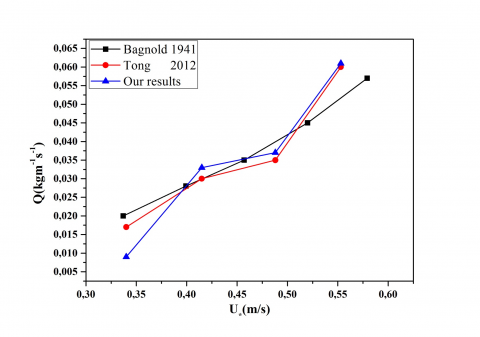Figure 3. Comparison of the masse flux with results of Bagnold and of Tong 

The sand-coupled wind velocities profiles for different values of the inlet velocity are shown in Figure 4. Note that the profiles undergone a braking effect caused by the inertia of the mass of the transported sand grains. It has been previously noted from experiments that the wind velocity profile does not perfectly follow the logarithmic law in this saltation layer  which was also observed in some numerical models , .

Figure 5 show the comparison between the inlet wind profile and the sand-coupled wind profile. It can be seen that the velocity profile near to the surface is modified by saltating particles the effective height of influence increases with rising inlet wind velocity because more energy is transferred to sand grains from the air and the grains can jump higher .  In the recent work of   discussed the particle velocity profile and found that it is insensitive to the wind shear stress and it was expressed as a function of the height and grain diameter. On another side in the present work; a sand-coupled wind velocity profile is showed and written as a function of the height. This function is dependent on both grain diameter and wind inlet velocity; this is due to the presence of the wind and grain in the sand-coupled wind. The shape of all the curves can be divided into two parts: The first part is for heights z> 0.05 m where the velocity profile follows a logarithmic function. The second part is for z <0.05m, where the velocity profile in the layer with saltation grains can be described using a linear function . This change appears in the so called “Transport layer”. Close to the bed; a layer whose depth is roughly corresponding to the Bagnold focuses height.  In the Transport layer; the sand-coupled wind velocity profile is found sensitive to the height as flowing:

$U(z)=U_{0}+\gamma z U$

With the average sleep velocity U0 and the shear rate $\gamma$ are functions of wind inlet velocity and the grain diameter:

$U_{0}(v)=C_{a} V$

$\gamma(V, d)=C_{b} V+C_{c} \sqrt{\frac{g}{d}}$

With the flowing dimensionless constant:

$C_{a}=0.034, C_{b}=4.53, C_{c}=3.83$

Since, velocity tends to increase rapidly at boundary layer, so concluding from the results that the boundary layer is the height where the velocity profile changes from linear to logarithmic trend. The velocity is an important element in studying sand vertical distribution and it’s an essential and basic parameter in the research of sand transport by wind.

## 4.png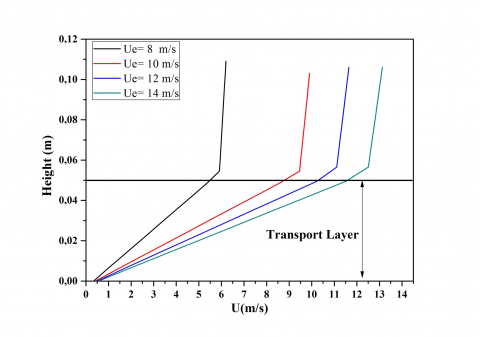Figure 4.  Variation of the   mean horizontal velocity with increasing height

## 5.png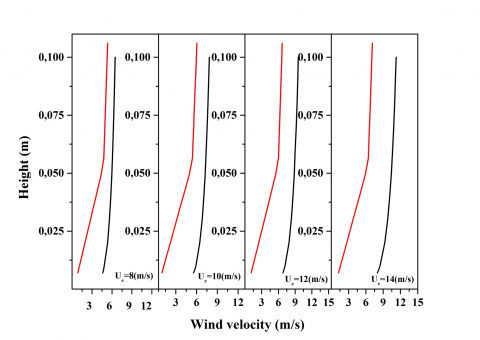Figure 5. Comparison of the inlet wind profile and sand-coupled wind profile at different wind velocity

Figure 6 shows the variation of the density with height for different velocities. Note that, near to the ground the maximum density increases with the rising inlet wind velocity. While it tends to zero for height values over 0.14 m, which is in compliance with the experimental results.

The sand flux is calculated with a relation between the density of the transported sand Eq(6) and the velocity of the mixture shown in Figure 4.

Figure 7 shows the evolution of the flux profile with the variation in wind velocity. The sand flux increases with wind velocity. In the experimental work of  the sand flux was measured from the height of 3cm. In the present work the sand flux profile can be calculated from the roughness height. Again, profiles follow two shapes: the first represents the profile near the surface in which the saltation flux increases with height; we note the existence of an inflection point where the maximum flow occurs, at a height of 0.045 m. This height is in the inside of the boundary layer. After the inflection point, the flux is reduced down to zero following a form of the exponential function. We conclude that the flux is expressed as a Gaussian distribution;  gave a name for this type of flux density: the peak-flow-profile pattern. In the theory of  shows that far the saltation of small particles in strong turbulence the saltation mass flux density profile is Gaussian distribution. Particle lift-off velocity and incident angle have an important role in the profile of saltation mass flux , . Modeling results of showed that far particles with same diameter. The simulated vertical sand flux decayed with Gaussian distribution with increasing height if the lift-off velocity and incident angle showed Gaussian distribution.

From results in this work, it can be concluded that; the lift-off velocity and incident angle have a Gaussian distribution for fine particle (0.063-0.08 mm).

## 6.png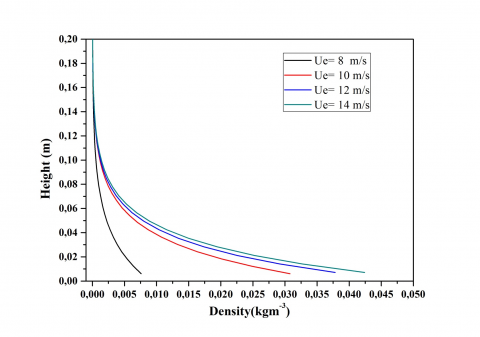Figure 6. Variation of density with increasing height

## 7.png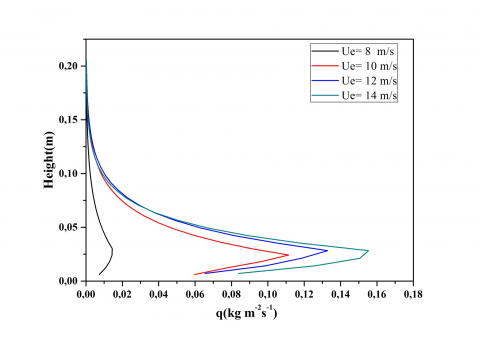Figure 7.  Variation of sand flux with increasing height

It is noted from the simulation results that the velocity increases accordingly as the height increases, consequently, the kinetic energy increases too. The curve of the energy has a peak at 0.055 m. Before this height the kinetic energy increased rapidly whereas after the peak it declined slowly. Although we have seen that the sand mass flux curve has decreased with the heights, it is seen that the presence of sand affects the shape of the turbulent kinetic energy curve. Figure 8 shows the development of energy curves with the variation of the wind velocity. All of them consist of two shapes that we can divide into two height intervals. The first one is about (0-0.05m), where the energy increases with the height; this increase is due to the rapid increase in wind velocity, and the uplift of the sand grains. The second is (0.05-0.20m) where the energy decreases, it is due to decrease in the amount of sand in the mixture air-sand. The energy is absorbed by the falling grains. It can be seen here that the thickness of the boundary layer is about 0.05m as found with the velocity curves.

## 8.png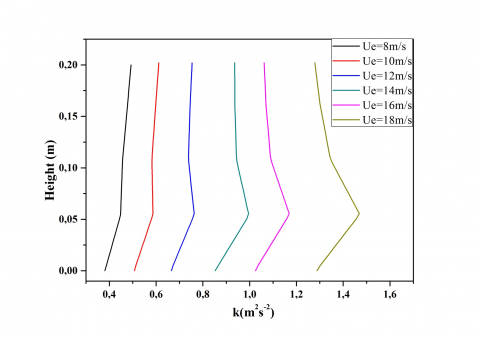Figure 8. Variation in the turbulent kinetic energy with increasing height

In this section the variation in sand flux and in turbulent kinetic energy are presented with increasing height. Satisfactory correlation cannot be found between the sand flux and energy in the boundary layer for lowest wind velocity, i.e. 8m/s. For velocity 10m/s to 14m/s it is observed that the sand flux and the pick of the turbulent kinetic energy are situated in the boundary layer, however, the two picks approach each other with increasing velocity.

## 9a.png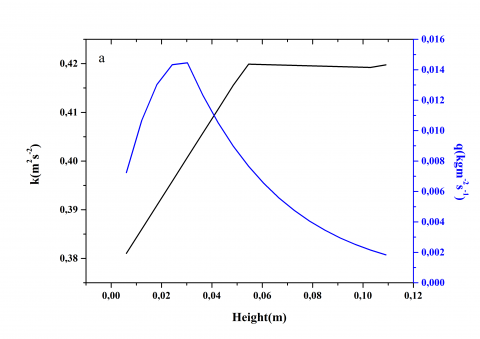## 9b.png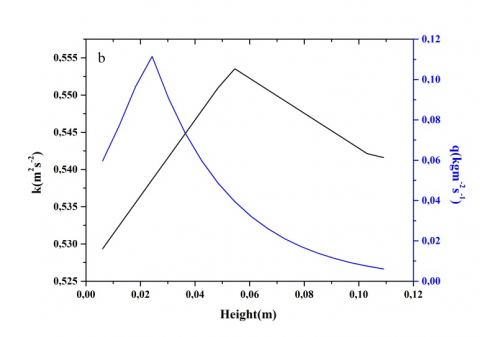## 9c.png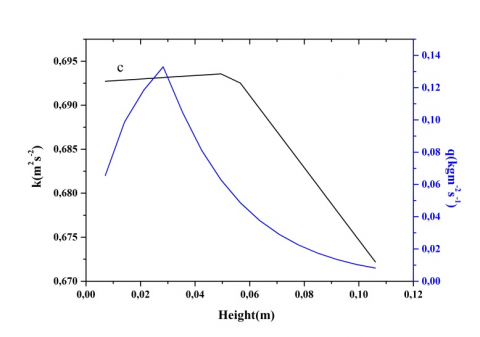## 9d.pngFigure 9. Comparison of the kinetic energy and the sand flow with increasing height (8, 10, 12 and 14m/s) respectively

The observation means that the turbulences causes grains to bounce and appears only when there is a big concentration of sand, but decrease of grains quantity in the air leads to decrease in the turbulence.

The turbulence eddy dissipation is the rate at which the velocity fluctuations dissipate.  The dissipation follows the shape of the exponential function $a e^{-z b}$ this explains the behavior of the energy curve. It can be seen that the dissipation took the largest value in the boundary layer, and then it decreases with the increasing height. Figure 10 also shows that the dissipation is linked to the wind velocity; moreover the velocity is greater more the dissipation takes high values in the boundary layer. With height, the dissipation tends to a one value for all velocities.

## 10.png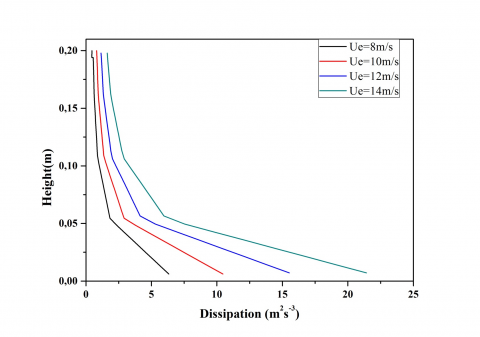Figure 10. Variation of dissipation with increasing height

5. Conclusions

The determination of quantities of sand displaced in time remains the main objective of all the previous studies on Aeolian transport. In addition to correlations emanating from experimental data, many models have been proposed for the last decades in order to acquire reliable Tools for the prediction of desertification progress. By the introduction of a statistical approach involving a distribution function gathering the dynamic properties of the flow and coupled with the equations of momentum. We succeeded in evaluating the quantities of sand transport and the results showed conformity with the experimental results. Even in the detail; the changing properties with height described very well the actual behavior either for sand density or for velocity and turbulence kinetic energy.

References

 G. Fécan, F., Marticorena, B., Bergametti, “Parametrization of the increase of the aeolian erosion threshold wind friction velocity due to soil moisture for arid and semi-arid areas,” Ann. Geophys., vol. 17, pp. 149–157, 1999.

 S. L. Namikas, “Field measurement and numerical modelling of aeolian mass flux distributions on a sandy beach,” Sedimentology, vol. 50, no. 2, pp. 303–326, 2003.

 R. A. Bagnold, The Physics of Blown Sand and Desert Dunes, Methuen. London, 1941.

 Y. Shao, “Physics and modelling of wind erosion,” Atmos. Oceanogr. Sci. Libr., vol. 37, p. 452, 2008.

 J. Shi, D. Yu, X. Ji and X. Ai, “Prediction of Fluvial sand body using the technique of frequency division interpretation,” vol. 2, no. 2, pp. 13–18, 2015.

 Z. Dong, X. Liu, H. Wang, A. Zhao and X. Wang, “The flux profile of a blowing sand cloud: A wind tunnel investigation,” Geomorphology, vol. 49, no. 3–4, pp. 219–230, 2003.

 L. Tan, W. Zhang, J. Qu, J. Du, D. Yin and Z. An, “Variation with height of aeolian mass flux density and grain size distribution over natural surface covered with coarse grains: A mobile wind tunnel study,” Aeolian Res., vol. 15, pp. 345–352, Dec. 2014.

 D. Tong and N. Huang, “Numerical simulation of saltating particles in atmospheric boundary layer over flat bed and sand ripples,” J. Geophys. Res., vol. 117, no. D16, p. D16205, 2012.

 L. Kang and D. Liu, “Numerical investigation of particle velocity distributions in aeolian sand transport,” Geomorphology, vol. 115, no. 1–2, pp. 156–171, 2010.

 W. Zhang, H. Liu, X. Du, Y. Yang and L. Shi, “Numerical and experimental research on performance of single-row finned tubes in air cooled power plants,” Int. J. Heat Technol., vol. 34, no. 1, pp. 137–142, 2016.

 S. Dupont, G. Bergametti, B. Marticorena and S. Simoëns, “Modeling saltation intermittency,” J. Geophys. Res. Atmos., vol. 118, no. 13, pp. 7109–7128, 2013.

 A. Valance, K. R. Rasmussen, A. Ould El Moctar and P. Dupont, “The physics of Aeolian sand transport,” Comptes Rendus Phys., vol. 1, pp. 1–13, 2015.

 H. J. Sauermann, G., Kroy, K., Herrmann, “Continuum saltation model for sand dunes,” Phys. Rev, vol. E 64, p. 031305, 2001.

 J. T. Jenkins, I. Cantat and A. Valance, “Continuum model for steady, fully developed saltation above a horizontal particle bed,” Phys. Rev. E, vol. 82, no. 2, p. 020301, 2010.

 T. Pähtz, A. Omeradˇ, M. V Carneiro, N. M. Ara and H. J. Herrmann, “Which length scale dominates saturation of aeolian sand transport ?,” Geophys. Res. Lett., no. 2, 2014.

 M. Lämmel, D. Rings and K. Kroy, “A two-species continuum model for aeolian sand transport,” New J. Phys., vol. 14, 2012.

 David C. Wilcox, “Turbulence modeling for CFD.” p. 477, 1993.

 B. E. Launder and D. B. Spalding, “The numerical computation of turbulent flows,” Comput. Methods Appl. Mech. Eng., vol. 3, no. 2, pp. 269–289, 1974.

 T. D. Ho, A. Valance, P. Dupont and a. Ould El Moctar, “Aeolian sand transport: Length and height distributions of saltation trajectories,” Aeolian Res., vol. 12, pp. 65–74, 2014.

 B. Andreotti, P. Claudin and O. Pouliquen, “Measurements of the aeolian sand transport saturation length,” Geomorphology, vol. 123, no. 3–4, pp. 343–348, 2010.

 Z. Dong, X. Liu, H. Wang and X. Wang, “Aeolian sand transport : a wind tunnel model,” Sediment. Geol., vol. 161, pp. 71–83, 20003.

 Z. S. Li, D. J. Feng, S. L. Wu, A. G. L. Borthwick and J. R. Ni, “Grain size and transport characteristics of non-uniform sand in aeolian saltation,” Geomorphology, vol. 100, no. 3–4, pp. 484–493, Aug. 2008.

 RP Owen, “Saltation of uniform grains in air,” J Fluid Mech, vol. 20, pp. 225–242, 1964.

 J. . Ni, Z. . Li and C. Mendoza, “Vertical profiles of aeolian sand mass flux,” Geomorphology, vol. 49, no. 3–4, pp. 205–218, 2003.

 P. K. Anderson, R. S., Haff, “Wind modification and bed response during saltation of sand in air,” Acta Mech., vol. Suppl., 1, pp. 21–52, 1991.

 A. Shao, Y., Li, “Numerical modelling of saltation in the atmospheric surface layer,” Bound. Layer Meteorol, vol. 91(2), 199, pp. 199–225, 1999.

 Y. Shao, “A similarity theory for saltation and application to aeolian mass flux,” Boundary-Layer Meteorol., vol. 115, no. 2, pp. 319–338, 2005.